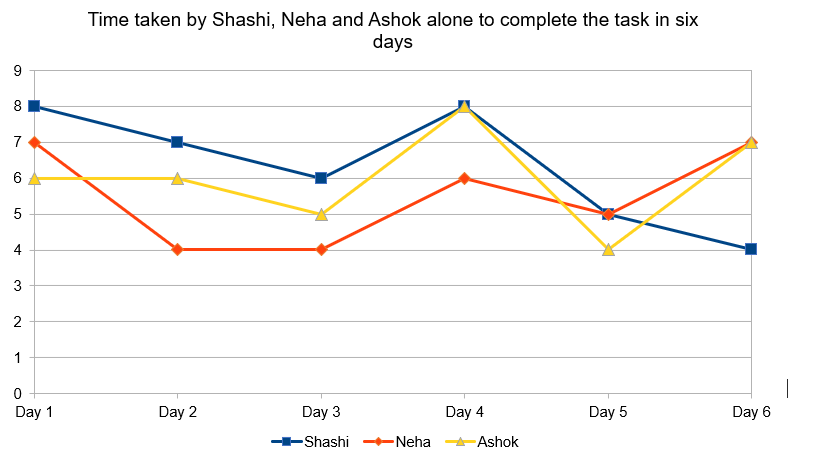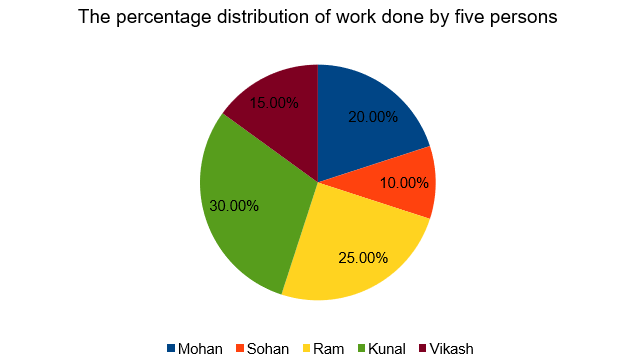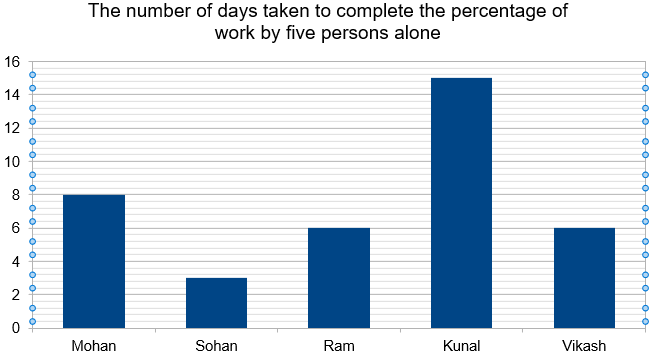# Data Interpretation Based on Time and Work | Concept and Practice Questions

In this article, we will talk about Data Interpretation based on Time and Work. In Data Interpretation, a large volume of data is organized and is represented into a compact and precise form which is easier to interpret than the raw data. Students are required to draw conclusions and inferences from comprehensive data presented numerically. The data is given in the form of graphs (pie chart, bar graph, line graph, etc), tables, or in the form of caselets.

Register Here to Take Oliveboard’s Free Mock Test

## 1. Introduction to Data Interpretation Based on Time and Work

Data Interpretation based on Time and work is a type of representation in which values of variables are represented in proportion with efficiency or number of days taken by men or women to complete the work or part of work done. This topic is very important as in the recent examinations we have seen this kind of DI being asked very frequently.

For more understanding, you can also watch the video on Data Interpretation based on Time and Work. In this video, he discussed questions that are based on Time and Work. To watch this video please click here.

Register on Oliveboard & Get Bank, SSC & RRB NTPC Free Mock Tests

## 2. Practice Questions for Data Interpretation Based on Time and Work

Let us understand Data Interpretation based on Work and Time with the following two examples:

### SET 1: 5 Questions

The following data shows the working hours for three employees (Shashi, Neha, and Ashok) in six days to complete a task. The working hours also indicates the time taken by them to complete the same task alone.Q1. What is the difference between the average of working hours of Shashi on Day 1 and Day 6 together and the average of working hours of Neha on Day 5 and Day 6 together?

(1) 4.5

(2) 2

(3) 4

(4) 3

(5) 0

Solution:

Average of working hours of Shashi on Day 1 and Day 6 together = (8 + 4)/2 = 6 hours

Average of working hours of Neha on Day 5 and Day 6 together = (5 + 7)/2 = 6 hours

Required difference = 6 – 6 = 0

Q2. On Day 2, If Shashi takes half the working hour time to complete a work while Neha takes equal time as Shashi, and Ashok takes 50% more time to complete the same work alone. What will be the ratio of their working efficiency to complete a work?

(1) 12: 18: 7

(2) 18: 18: 7

(3) 9: 9: 7

(4) 12: 12: 7

(5) None of these

Solution:

Shashi takes =7/2 hours

Neha takes = Shashi = 7/2 hours

And Ashok takes = 150/100 * 6 = 9 hours

So, ratio of their efficiency = 2/7: 2/7: 1/9 = 18: 18: 7

Q3. Working hours on Day 5 by Shashi, Neha, and Ashok are the time taken by them to complete a work alone. How much time will they take to complete thrice the same work working together?

(1) 4(8/13) hours

(2) 3(9/13) hours

(3) 4(7/13) hours

(4) 7(7/13) hours

(5) None of these

Solution:

Time taken by them to complete thrice of the work = 3 * 1/(1/5 + 1/5 + ¼) = 4(8/13) hours

Q4. On Day 4, Shashi was supposed to complete the task on time. Due to health issues, he stopped when only one-third of the task was completed. Half of the remaining work was completed by Neha alone and the rest by Ashok alone. What is the total time taken by them to complete the task?

(1) 7 hours

(2) 7(1/3) hours

(3) 6(1/2) hours

(4) 8 hours

(5) None of these

Solution:

On Day 4:

One-third of the work done by Shashi = 8/3 hours

One-third of the work done by Neha = 6/3 = 2 hours

And rest one-third of the work done by Ashok = 8/3 hours

So, total time = 8/3 + 2 + 8/3 = 7(1/3) hours

Q5. What is the ratio of total working hours of Shashi and Ashok together throughout the period of six days to that of Neha’s throughout the period of six days?

(1) 71: 33

(2) 77: 34

(3) 74: 33

(4) 75: 32

(5) None of these

Solution:

The  total working hour of Shashi and Ashok together throughout the six days = 8 + 6 + 7 + 6 + 6 + 5 + 8 + 8 + 5 + 4 + 4 + 7 = 74 hours

And total working hour of Neha throughout the six days = 7 + 4 + 4 + 6 + 5 + 7 = 33 hours

So, required ratio = 74: 33

### SET 2: 5 Questions

Study the data given below carefully and answer the following questions:

The Pie Chart given below shows the percentage distribution of work done by five persons (Mohan, Sohan, Ram, Kunal, Vikash):The Bar graph given below shows the number of days taken to complete the percentage of work (which is mentioned in the above pie chart) by these five persons alone:Q6. What is the ratio of efficiency of Ram and Vikash?

(1) 4: 5

(2) 5: 3

(3) 4: 3

(4) 5: 4

(5) None of these

Solution:

So, required ratio = 1/24: 1/40 = 5: 3

Q7. If Garima is 20% more efficient than Sohan, then Mohan and Garima together can complete the whole work in how many days?

(1) 1000/29 days

(2) 1000/31 days

(3) 100/31 days

(4) 100/29 days

(5) None of these

Solution:

Garima alone can complete the whole work in = 300 * 100/120 = 250 days

So, Mohan and Garima together can complete the whole work in = 1/(1/250 + 1/40) = 1000/29 days

Q8. What is the difference between the time taken by Sohan and Vikash together to complete the whole work to the time taken by Kunal and Mohan to complete the whole work?

(1) 2000/149 days

(2) 2000/151 days

(3) 2000/153 days

(4) 2000/157 days

(5) None of these

Solution:

The  time taken by Sohan and Vikash together to complete the whole work = 1/(1/300 + 1/40) = 600/17 days

And  time taken by Kunal and Mohan to complete the whole work = 1/(1/50 + 1/40) = 200/9 days

So, required difference = 600/17 – 200/9 = 2000/153 days

Q9. If Parul takes 10 days less than Kunal and Annu takes 6 days more than Ram to complete the whole work, then in how many days, Parul, Annu and Mohan together can complete the whole work?

(1) 10 days

(2) 12 days

(3) 16 days

(4) 15 days

(5) None of these

Solution:

Parul alone can complete the whole work in = 50 – 10 = 40 days

Annu alone can complete the whole work in = 24 + 6 = 30 days

Required days = 1/(1/40 + 1/30 + 1/40) = 12 days

Q10. If Ashok takes 20 days more than Mohan and Pratima is 20% less efficient than Vikash, then time taken by Ashok to complete the whole work is what percentage of the time taken by Pratima to complete the whole work?

(1) 100%

(2) 120%

(3) 110%

(4) 140%

(5) 90%

Solution:

Ashok alone can complete the whole work in = 20 + 40 = 60 days

Pratima alone can complete the whole work in = 100/80 * 40 = 50 days

So, required % = 60/50 * 100 = 120%

Common Solution:

20% of the work done by Mohan in = 8 days

So, Whole work done by Mohan in = 100/20 * 8 = 40 days

10% of the work done by Sohan in = 3 days

So, whole work done by Sohan in = 30 * 100/10 = 300 days

25% of the work done by Ram in = 6 days

So, whole work done by Ram in = 100/25 * 6 = 24 days

30% of the work done by Kunal in = 15 days

So, whole work done by Kunal in = 100/30 * 15 = 50 days

And 15% of the work done by Vikash in = 6 days

So, Whole work done by Vikash in = 100/15 * 6 = 40 days

Read Here to Find Tips and Tricks to Increase your Calculation Speed

Want to solve Pie Charts in seconds?- Know how!

Attempt free Numerical Ability Section Test Now

That is all from us in this article. We wish you all the best for your journey throughout! You will surely make it!

Always choose the best for your preparation- Choose Oliveboard!

### 1 thought on “Data Interpretation Based on Time and Work | Concept and Practice Questions”

1.Mam/sir ,DIs mains level imp topics upload kijiye .